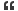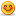# Thread: Noon + soon + moon = june

1. ## Noon + soon + moon = june

Find the values of each of the alphabets.
N o o n
s o o n +
m o o n
----------
j u n e

hint: each one of alphabets stands for distinct number between 0 and 9

-- james.Reply With Quote

2. ##Re: Noon + soon + moon = juneOriginally Posted by jamesravidFind the values of each of the alphabets.
N o o n
s o o n +
m o o n
----------
j u n e

hint: each one of alphabets stands for distinct number between 0 and 9

-- james.

First one If we use N and n are same than the answer is ....

u=3, o=4, N=n=2, e=6, s=5, m=0, j=8.

If we use N and n are different then the answer is....

u=3, 0=4, n=2, e=6, N=0, s=7, m=1, j=9.

Am i correct...?Reply With Quote

3. ## Re: Noon + soon + moon = june

Actually i forgot to add one more condition. The condition is that most significant digit should not be 0.

Both N and n are same. If we include the above condition then values of m is 1 and j is 9. Any way your answer is perfect. If possible leave the solution here. It will be very useful to othersReply With Quote

4. ##Re: Noon + soon + moon = juneOriginally Posted by jamesravidFind the values of each of the alphabets.
N o o n
s o o n +
m o o n
----------
j u n e

hint: each one of alphabets stands for distinct number between 0 and 9

-- james.
Hello James
Can you pls give the explaination i have got n=2 but i dont understand why m should be 1Reply With Quote

5. ## Re: Noon + soon + moon = june

hi psuresh

how did you assign this statement as u=3, o=4, N=n=2, e=6, s=5, m=0, j=8 is there any technical indication to assign the numbers

Thanks
DeepasreeReply With Quote

6. ## Re: Noon + soon + moon = june

noon 8228
soon 0228
moon 1228
----- -----
june 9684
----- -----Reply With Quote

7. ## Re: Noon + soon + moon = june

u r not correct actual value is also.....
N=2
O=4
J=9
S=5
U=3
M=1
E=6Reply With Quote

8.

####Posting Permissions

• You may not post new threads
• You may not post replies
• You may not post attachments
• You may not edit your posts
•